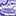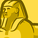# QlikView App Dev

Discussion Board for collaboration related to QlikView App Development.

Announcements
Join us at the Cloud Data and Analytics Tour! REGISTER TODAY
cancel
Showing results for
Search instead for
Did you mean:Contributor

## How to create if statement to change text to number?

Hi,

I'm trying to write an if statement which changes a text rating to a numeric one.

For example;

changing 'Highly Satisfied' to 1

Thanks for any assistance.

1 Solution

Accepted SolutionsMaster

If(Rating = 'Highly Satisfied', 1) would work here. If you have a small number of options, just nest a few If() statements. If you have a large number of options, I'd suggest adding a translation/mapping table in script.

Note that if you do this in your script, you can use Dual() to create one column that holds both the text and numeric value, e.g.

Dual(Rating, if(Rating='Highly Satisfied',1)) as DualRating

You can then use this value in display (where it will be text) or in mathematical formulas (where it will behave as a number)

2 RepliesMaster

If(Rating = 'Highly Satisfied', 1) would work here. If you have a small number of options, just nest a few If() statements. If you have a large number of options, I'd suggest adding a translation/mapping table in script.

Note that if you do this in your script, you can use Dual() to create one column that holds both the text and numeric value, e.g.

Dual(Rating, if(Rating='Highly Satisfied',1)) as DualRating

You can then use this value in display (where it will be text) or in mathematical formulas (where it will behave as a number)Specialist

you cam also try with pick match function

=Pick(Match([your_Field_name],'Highly Satisfied','medium Satisfied','low Satisfied'),1,2,3)

or

=concat( DISTINCT Pick(Match([your_Field_name],'Highly Satisfied','medium Satisfied','low Satisfied'),1,2,3) ,',')